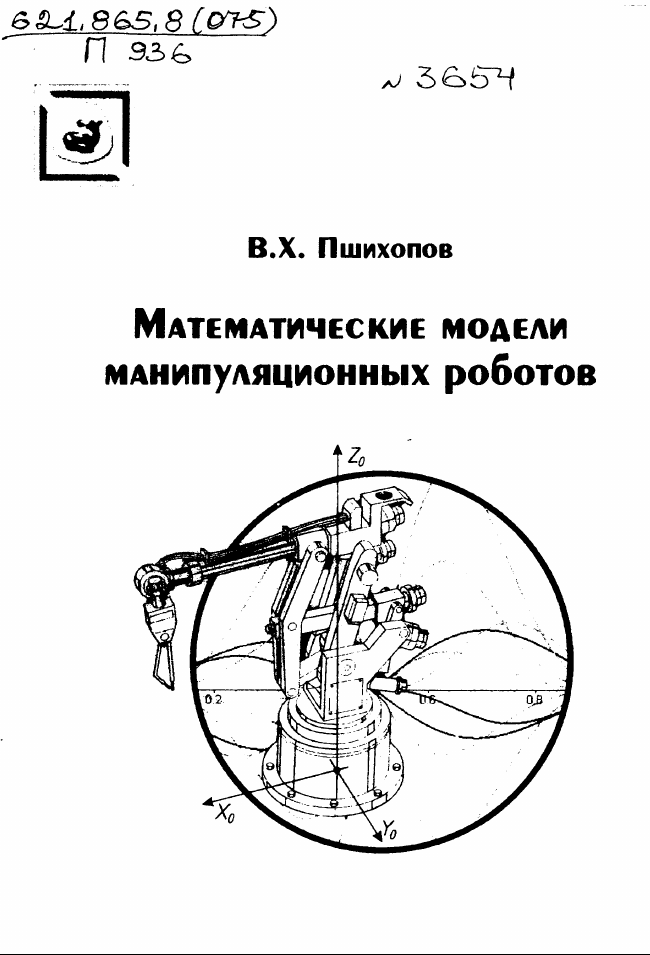This study guide presents formalized procedures of creating mathematical models of manipulating robots. The algorithms of solving the kinematic tasks of open kinematic chains are presented together with derivations of manipulator dynamics equations. The models of manipulator robots dynamics are presented in different spaces accounting for drives’ models and possible interactions with the environments. Examples of writing mathematical models are given.